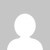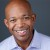# T-SQL: Calculating number of weekdays between two dates

Tonight someone tweeted trying to find a ColdFusion or SQL solution to counting the number of weekdays (excluding Saturdays and Sundays) between two dates.  Most of the solutions online involving looping over the date range and adding to a counter if that iteration of the loop is a weekday.

That’s fine for limited, but probably not if you need to test dates that span many years or need to run the function 1,000s of times.  Anyway, here’s a SQL Server UDF solution.  It’s late, so hopefully the comments in the code suffice.  It should be straightforward to rewrite this in ColdFusion.

This is an improved version of the solution presented here.  That solution fails when the timespan is less than 7 days long and contains at least one weekend day.

```create function [dbo].[dateDiffWeekdays] (
@startdaytime DATETIME;
@enddaytime DATETIME;
)
returns int
as
begin

-- Strip Times

-- handle end conditions
DECLARE @firstWeekDayInRange datetime, @lastWeekDayInRange datetime;
select @firstWeekDayInRange =
case
-- If Saturday, add two days
when datepart(dw,@start) = 7 then dateadd(day,2,@start)
-- If Sunday, add one day
when datepart(dw,@start) = 1 then dateadd(day,1,@start)
else @start
end

select @lastWeekDayInRange =
case
-- If Saturday, substract one day
when datepart(dw,@end) = 7 then dateadd(day,-1,@end)
-- If Sunday, substract two days
when datepart(dw,@end) = 1 then dateadd(day,-2,@end)
else @end
end

-- add one day to answer (to count Friday) if enddate was on a weekend
if @end != @lastWeekDayInRange

case
-- triggered if start and end date are on same weekend
when dateDiff(day,@firstWeekDayInRange,@lastWeekDayInRange) < 0 then (@answer * -1)
-- otherwise count the days and substract 2 days per weekend in between dates
else (DateDiff(day, @firstWeekDayInRange, @lastWeekDayInRange) - DateDiff(week, @firstWeekDayInRange, @lastWeekDayInRange)*2)
end

end

-- Call created function
select dbo.dateDiffWeekdays('6/1/2009','6/13/2009')```

### 4 thoughts on “T-SQL: Calculating number of weekdays between two dates”

1.Hi. I like the way you write. Will you post some more articles?

2.is this “datefirst” independent?

3.It’s not @@datefirst independent. I’m assuming that Sunday is the first day of the week when using datepart to test whether or not the start and end dates fall on the weekend.

4.Use this technique instead (from http://stackoverflow.com/questions/252519/count-work-days-between-two-dates)

DECLARE @StartDate DATETIME
DECLARE @EndDate DATETIME
SET @StartDate = ‘2008/10/01’
SET @EndDate = ‘2008/10/31’

SELECT
(DATEDIFF(dd, @StartDate, @EndDate) + 1)
-(DATEDIFF(wk, @StartDate, @EndDate) * 2)
-(CASE WHEN DATENAME(dw, @StartDate) = ‘Sunday’ THEN 1 ELSE 0 END)
-(CASE WHEN DATENAME(dw, @EndDate) = ‘Saturday’ THEN 1 ELSE 0 END)

Scroll to Top